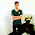Addition, subtraction, multiplication, and transpose on the matrix

If A and B are any two matrix of the same size, then the number of A + B is the matrix obtained by adding the corresponding entries in the two matrix together. Different size matrix cannot be added.

Example 1.

because the order size is different, namely A and B measuring 2 x 2 while C is 2 x 3, A + C and B + C are not defined.

If B is any matrix, then -B will declare the product of multiplication (-1) B. If A and B are two matrix of the same size, then A - B is defined as the number of A + (-B) = A + (- 1)

Example 2.
Review the matrix

Scalar Multiplication of Matrix

If A is a matrix and c is a scalar, then the product cA is a matrix obtained by multiplying each entry from A by c.

Example 3.
Note:
Some laws for scalar addition and multiplication: If A, B, C are equal sized matrix, and c scalar is:
1. A = B = B + A (commutative)
2. (A + B) + C = A + (B + C) (associative)
3. c (A + B) = cA + cB (distributive)
4. There is always a matrix D such that A + D = B

Multiplication of Matrix

Above we have defined scalar matrix multiplication. The following will be explained about how we multiply two matrix together. At matrix multiplication AB, then the matrix A we call the first matrix and B the second matrix.

Multiplication Matrix Requirements: Number of number of first matrix columns = number of second row matrix.

Definition of Multiplication of Matrix: If A is the matrix m x r and B is a matrix r x n, then the product of AB is the matrix m x n whose entries are determined as follows. To search for entries in row i and column i of AB, select row i from matrix A and column j from matrix B. Multiply the corresponding entries from the rows and columns together and then add the resulting multiplication results.

Example 4.

Defined BA with size (2 x 3)

But AB is undefined.

The definition of matrix multiplication requires that the number of rows of factor A must be the same as the number of columns of the second factor B, which forms the product of AB. If this condition is not met, the multiplication results cannot be defined.

Matrix multiplication has an important application to the system of linear equations. Review a system consisting of m linear equations in unknown numbers.

Because two matrix are declared equal if and only if the corresponding entries are the same, we can replace the m equation in this system with a single matrix equation.

The m x 1 matrix on the left hand side of the equation can be written as a product of multiplication

So we mark these matrix in a row with A, X, and B, so the system m of the original equation in n unknown numbers has been replaced by a single matrix equation,

AX=B

he A matrix in this equation is called the coefficient matrix for the system.
Some laws on matrix multiplication:

If A, B, C matrix meet the required matrix multiplication requirements, then:
1. A (B + C) = AB + AC, (B + C) A = BA + CA, fulfills distributive law.
2. A (BC) = AB (C), fulfills associative law.
3. Non-commutative multiplication, AB ≠ BA.
4. If AB = 0 (zero matrix), which is a matrix with all its elements = 0, the possibilities are: (i) A = 0 and B = 0, (ii) A = 0 or B = 0, (iii) A ≠ 0 and B ≠ 0
5. If AB = AC is not necessarily B = C

Transposes From A Matrix

If A is any matrix m x n, then transpose A is expressed by At and is defined by the matrix n x m whose first column is the first row of A, the second column is the second row of A, so the third column is the third row from A onwards.

Example 5.

3 Responses to "Addition, subtraction, multiplication, and transpose on the matrix"

1.So simple.. its made me understand about matrix

2.Saya sulit untuk mengerti tentang matriks hehehehe

3.Saya sulit untuk mengerti tentang matriks hehehehe